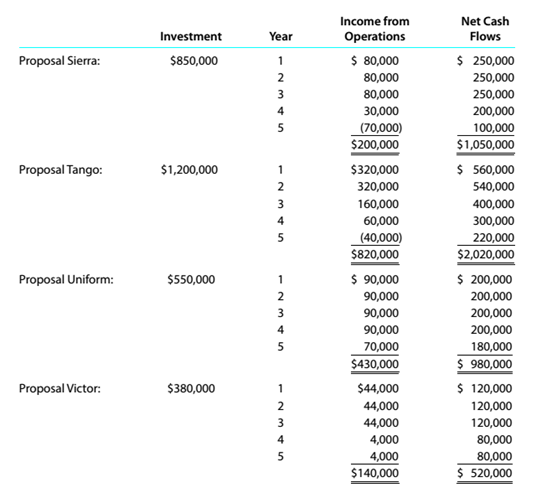Chapter 15, Problem 15.6.3P

Chapter
Section
Textbook Problem

Capital rationing decision involving four proposalsKopecky Industries Inc. is considering allocating a limited amount of capital investmentfunds among four proposals. The amount of proposed investment, estimated income fromoperations, and net cash flow for each proposal are as follows:The company’s capital rationing policy requires a maximum cash payback period of three years. In addition, a minimum average rate of return of 12% is required on all projects. 1f the preceding standards are met, the net present value method and present value indexes are used to rank the remaining proposals. InstructionsUsing the following format, summarize the results of your computations in parts (I) and(2). By placing the calculated amounts in the first two columns on the left and by placinga check mark in the appropriate column to the right,analysis which proposals should beaccepted for further analysis and which should be rejected.To determine

Concept Introduction:

Payback Period:

Payback period is the period in which the project recovers its initial cost of the investment. It can be calculated by dividing the initial investment by the annual cash inflow from the project.

ARR:

Accounting Rate of Return (ARR) is the rate of return earned on the investment made in a project. ARR is calculated by dividing the Average Accounting profits by Average Investment.

The formula to calculate ARR is as follows:

ARR= Average Accounting profitsAverage Investment

To Indicate:

The decision for each proposal on the basis of previous two analyses

Explanation

The decision for each proposal on the basis of previous two analyses is taken as follows:

All the projects with either higher than 3 years payback period or lower than 12% Average rate of return are rejected.

 Cash Payback Period Average Rate of Return Accept for Further Analysis Reject Proposal Sierra 3.50 4.7% ✓ Proposal Tango 2...

Still sussing out bartleby?

Check out a sample textbook solution.

See a sample solution

The Solution to Your Study Problems

Bartleby provides explanations to thousands of textbook problems written by our experts, many with advanced degrees!

Get Started

How can sensitivity analysis be used in conjunction with CVP analysis?

Managerial Accounting: The Cornerstone of Business Decision-Making

Should an economic model describe reality exactly?

Principles of Microeconomics (MindTap Course List)

PREFERRED STOCK VALUATION Ezzell Corporation issued perpetual preferred stock with a 10% annual dividend. The s...

Fundamentals of Financial Management, Concise Edition (with Thomson ONE - Business School Edition, 1 term (6 months) Printed Access Card) (MindTap Course List)